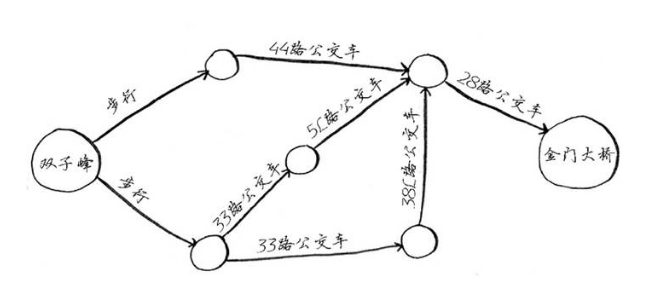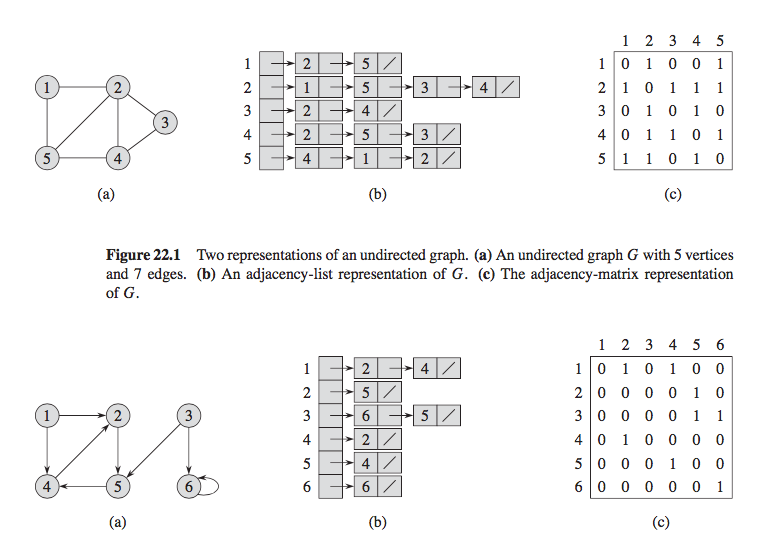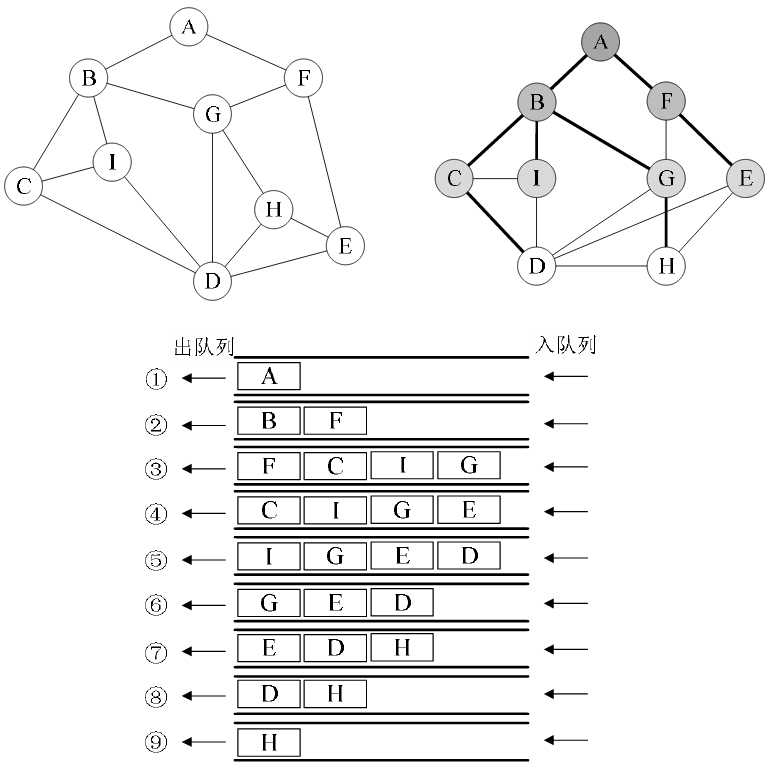# 什么是图？# 图的表示• 邻接表法：对于每个图中的点，将它的邻居放到一个链表里
• 邻接矩阵：对于 n 个点，构造一个 n * n 的矩阵，如果有从点 i 到点 j 的边，就将矩阵的位置 matrix[i][j] 置为 1.

# 图的遍历

• DFS: Depdth First Search，深度优先搜索

### BFS

BFS 类似于树的层序遍历，从第一个节点开始，先访问离 A 最近的点，接着访问次近的点。我们先来构造一个图：

graph = {
'A': ['B', 'F'],
'B': ['C', 'I', 'G'],
'C': ['B', 'I', 'D'],
'D': ['C', 'I', 'G', 'H', 'E'],
'E': ['D', 'H', 'F'],
'F': ['A', 'G', 'E'],
'G': ['B', 'F', 'H', 'D'],
'H': ['G', 'D', 'E'],
'I': ['B', 'C', 'D'],
}


# -*- coding: utf-8 -*-

from collections import deque

GRAPH = {
'A': ['B', 'F'],
'B': ['C', 'I', 'G'],
'C': ['B', 'I', 'D'],
'D': ['C', 'I', 'G', 'H', 'E'],
'E': ['D', 'H', 'F'],
'F': ['A', 'G', 'E'],
'G': ['B', 'F', 'H', 'D'],
'H': ['G', 'D', 'E'],
'I': ['B', 'C', 'D'],
}

class Queue(object):
def __init__(self):
self._deque = deque()

def push(self, value):
return self._deque.append(value)

def pop(self):
return self._deque.popleft()

def __len__(self):
return len(self._deque)

def bfs(graph, start):
search_queue = Queue()
search_queue.push(start)
searched = set()
while search_queue:   # 队列不为空就继续
cur_node = search_queue.pop()
if cur_node not in searched:
yield cur_node
for node in graph[cur_node]:
search_queue.push(node)

print('bfs:')
bfs(GRAPH, 'A')
"""
bfs:
A
B
F
C
I
G
E
D
H
"""### DFS

DFS_SEARCHED = set()

def dfs(graph, start):
if start not in DFS_SEARCHED:
print(start)
for node in graph[start]:
if node not in DFS_SEARCHED:
dfs(graph, node)

print('dfs:')
dfs(GRAPH, 'A')  # A B C I D G F E H



# 思考题

• DFS 中我们使用到了递归，请你用栈来改写这个函数？（代码里有答案，我建议你先尝试自己实现）